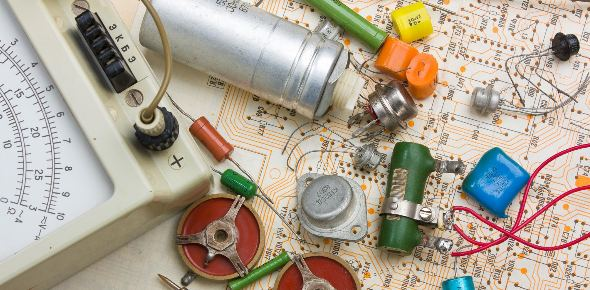# 2.1.4 Thinking Logically

13 QuestionsSettingsQuiz for Component 2 - 2. 1. 4 Thinking Logically

Related Topics
• 1.
6 < 10
• A.

True

• B.

False

• 2.
What is this logic symbol?
• A.

Less Than

• B.

Equal To

• C.

Greater Than

• D.

Not Equal To

• 3.
TRUE and TRUE = [BLANK]
• 4.
TRUE and [BLANK] = FALSE
• 5.
What would be the outcome of this code if A = TRUE and B = FALSE
• A.

True

• B.

False

• 6.
Select the correct pseudocode for this problem:Mary wants a laptop with at least 8 GB Ram and a graphics card and no sound card
• A.

IF(ram < 8) AND graphicsCard = True AND soundCard = False

• B.

IF(ram > 8) AND graphicsCard = True AND soundCard = True

• C.

IF(ram > 8) AND graphicsCard = False AND soundCard = False

• D.

IF(ram > 8) AND graphicsCard = True AND soundCard = False

• 7.
6 [BLANK] 10 = FALSE
• 8.
1 [BLANK] 2 = TRUE
• 9.
7 [BLANK] 7 = TRUEHINT: IT'S NOT =
• 10.
((TRUE AND TRUE) OR (FALSE AND FALSE)) AND (FALSE OR TRUE) = [BLANK]
• 11.
6 x 9 < 37 = [BLANK]